Search by Topic

Resources tagged with Interactivities similar to Ding Dong Bell Interactive:

Filter by: Content type:
Age range:
Challenge level:

There are 96 results

Broad Topics > Information and Communications Technology > InteractivitiesDing Dong Bell Interactive

Age 16 to 18 Challenge Level:

Try ringing hand bells for yourself with interactive versions of Diagram 2 (Plain Hunt Minimus) and Diagram 3 described in the article 'Ding Dong Bell'.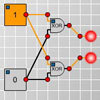Simple Counting Machine

Age 11 to 16 Challenge Level:

Can you set the logic gates so that the number of bulbs which are on is the same as the number of switches which are on?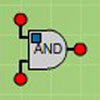Circuit Maker

Age 11 to 18 Challenge Level:

Investigate how logic gates work in circuits.Squaring the Rectangle

Age 14 to 18 Challenge Level:

Can you find a way to turn a rectangle into a square?Proof Sorter - Sum of an AP

Age 16 to 18 Challenge Level:

Use this interactivity to sort out the steps of the proof of the formula for the sum of an arithmetic series. The 'thermometer' will tell you how you are doingNine Colours

Age 11 to 16 Challenge Level:

Can you use small coloured cubes to make a 3 by 3 by 3 cube so that each face of the bigger cube contains one of each colour?Spinners Environment

Age 5 to 18 Challenge Level:

A tool for generating random integers.Loci Resources

Age 14 to 16 Challenge Level:

This set of resources for teachers offers interactive environments to support work on loci at Key Stage 4.Excel Interactive Resource: Fraction Multiplication

Age 11 to 16 Challenge Level:

Use Excel to explore multiplication of fractions.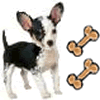Ratio Pairs 3

Age 11 to 16 Challenge Level:

Match pairs of cards so that they have equivalent ratios.Cops and Robbers

Age 7 to 16 Challenge Level:

Can you find a reliable strategy for choosing coordinates that will locate the robber in the minimum number of guesses?Cushion Ball Interactivity

Age 14 to 16 Challenge Level:

The interactive diagram has two labelled points, A and B. It is designed to be used with the problem "Cushion Ball"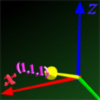Tracking Points

Age 14 to 16 Challenge Level:

Can you give the coordinates of the vertices of the fifth point in the patterm on this 3D grid?Excel Interactive Resource: Interactive Division

Age 11 to 16 Challenge Level:

Use an Excel to investigate division. Explore the relationships between the process elements using an interactive spreadsheet.Colour in the Square

Age 7 to 16 Challenge Level:

Can you put the 25 coloured tiles into the 5 x 5 square so that no column, no row and no diagonal line have tiles of the same colour in them?Proof Sorter - the Square Root of 2 Is Irrational

Age 16 to 18 Challenge Level:

Try this interactivity to familiarise yourself with the proof that the square root of 2 is irrational. Sort the steps of the proof into the correct order.Fractions and Percentages Card Game

Age 11 to 16 Challenge Level:

Match the cards of the same value.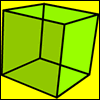Cubic Rotations

Age 14 to 16 Challenge Level:

There are thirteen axes of rotational symmetry of a unit cube. Describe them all. What is the average length of the parts of the axes of symmetry which lie inside the cube?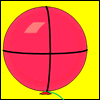Mesh

Age 16 to 18 Challenge Level:

A spherical balloon lies inside a wire frame. How much do you need to deflate it to remove it from the frame if it remains a sphere?Power Match

Age 16 to 18 Challenge Level:

Can you locate these values on this interactive logarithmic scale?Countdown Fractions

Age 11 to 16 Challenge Level:

Here is a chance to play a fractions version of the classic Countdown Game.White Box

Age 7 to 18 Challenge Level:

This game challenges you to locate hidden triangles in The White Box by firing rays and observing where the rays exit the Box.Interactive Workout - Mathmo

Age 16 to 18 Short Challenge Level:

Mathmo is a revision tool for post-16 mathematics. It's great installed as a smartphone app, but it works well in pads and desktops and notebooks too. Give yourself a mathematical workout!Prime Counter

Age 16 to 18 Challenge Level:

A short challenge concerning prime numbers.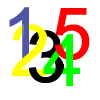Complex Countdown

Age 16 to 18 Challenge Level:

Play a more cerebral countdown using complex numbers.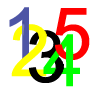Matrix Countdown

Age 16 to 18 Challenge Level:

Play countdown with matricesRed or Black Spinner

Age 3 to 16 Challenge Level:

A simple spinner that is equally likely to land on Red or Black. Useful if tossing a coin, dropping it, and rummaging about on the floor have lost their appeal. Needs a modern browser; if IE then at. . . .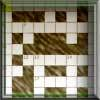Crossword 1

Age 16 to 18 Challenge Level:

A mathematically themed crossword.Factors and Multiples Game

Age 7 to 16 Challenge Level:

A game in which players take it in turns to choose a number. Can you block your opponent?Excel Interactive Resource: Long Multiplication

Age 11 to 16 Challenge Level:

Use an Excel spreadsheet to explore long multiplication.Excel Interactive Resource: the up and Down Game

Age 11 to 16 Challenge Level:

Use an interactive Excel spreadsheet to explore number in this exciting game!Euclid's Algorithm I

Age 16 to 18

How can we solve equations like 13x + 29y = 42 or 2x +4y = 13 with the solutions x and y being integers? Read this article to find out.Percentages for Key Stage 4

Age 14 to 16 Challenge Level:

A group of interactive resources to support work on percentages Key Stage 4.Excel Interactive Resource: Number Grid Functions

Age 11 to 16 Challenge Level:

Use Excel to investigate the effect of translations around a number grid.Solving with Euclid's Algorithm

Age 14 to 18 Challenge Level:

A java applet that takes you through the steps needed to solve a Diophantine equation of the form Px+Qy=1 using Euclid's algorithm.Excel Interactive Resource: Haitch

Age 11 to 16 Challenge Level:

An Excel spreadsheet with an investigation.Excel Interactive Resource: Fraction Addition & Fraction Subtraction

Age 11 to 16 Challenge Level:

Use Excel to practise adding and subtracting fractions.Factors and Multiples - Secondary Resources

Age 11 to 16 Challenge Level:

A collection of resources to support work on Factors and Multiples at Secondary level.Excel Interactive Resource: Multiples Chain

Age 11 to 16 Challenge Level:

Use an interactive Excel spreadsheet to investigate factors and multiples.Excel Interactive Resource: Equivalent Fraction Bars

Age 11 to 16 Challenge Level:

A simple file for the Interactive whiteboard or PC screen, demonstrating equivalent fractions.Tower Rescue

Age 11 to 18 Challenge Level:

Help the bee to build a stack of blocks far enough to save his friend trapped in the tower.Quick Route

Age 16 to 18 Challenge Level:

What is the quickest route across a ploughed field when your speed around the edge is greater?Changing Places

Age 14 to 16 Challenge Level:

Place a red counter in the top left corner of a 4x4 array, which is covered by 14 other smaller counters, leaving a gap in the bottom right hand corner (HOME). What is the smallest number of moves. . . .Square It

Age 11 to 16 Challenge Level:

Players take it in turns to choose a dot on the grid. The winner is the first to have four dots that can be joined to form a square.Got a Strategy for Last Biscuit?

Age 11 to 16 Challenge Level:

Can you beat the computer in the challenging strategy game?A Tilted Square

Age 14 to 16 Challenge Level:

The opposite vertices of a square have coordinates (a,b) and (c,d). What are the coordinates of the other vertices?Cyclic Triangles

Age 16 to 18 Challenge Level:

Make and prove a conjecture about the cyclic quadrilateral inscribed in a circle of radius r that has the maximum perimeter and the maximum area.Connect Three

Age 11 to 16 Challenge Level:

Can you be the first to complete a row of three?Balances and Springs

Age 14 to 16 Challenge Level:

Balancing interactivity with springs and weights.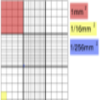Using the Haemocytometer

Age 14 to 16 Challenge Level:

Practise your skills of proportional reasoning with this interactive haemocytometer.